# Circles and Euler spiral (repost from general math)

• Mario

#### Mario

Hi,
i have this problem..., giving two circle (example radius 1 = 500 units, radius 2 = 200 units, distance between centers = 275.73 units) find Euler Spiral (aka Cornu spiral, aka Clothoid https://en.wikipedia.org/wiki/Euler_spiral) tangent giving circle (unknown tangent points).
For this problem are two mirrored Euler spiral as solution with length = 450 units.
Problem so simply to explain but not so simply to find solution...
Many thanks for help...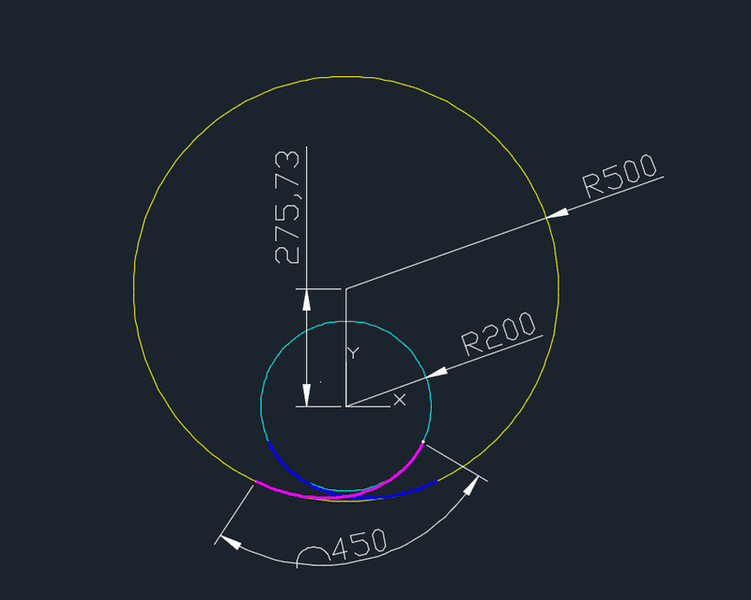Hello again, Mario, andagain, too

Well, we are making progress. There is a problem statement and the link gives a bunch of equations. So we can claim 1 and 2 below are filled in.
All we have to do now is make a start with 3 !

## The Attempt at a Solution

[/B]
Oh, and: don't erase the template (or was your thread moved here by a moderator?)

For a start: how did you find the 275.73 ?

## Homework Statement

[/B]
known data:
center of circle 1 (xO1,yO1) and radius of circle = R1
center of circle 2 (xO2,yO2) and radius of circle = R2

unknown data:
lenght of spiral (magenta and blue) L=?
tangent point of spiral on circle 1 (xA, yA) = ?
tangent point of spiral on circle 2 (xB, yB) = ?

## Homework Equations

Particular declination of problem are resolved with below equation, but in this resolution know data are:
R1, R2, L, xA=zero, yA=zero, xO1=zero, yO1=R1, line(O1-A) is orthogonal to x axes
calculated data are:
xO2, yO2, xB, yB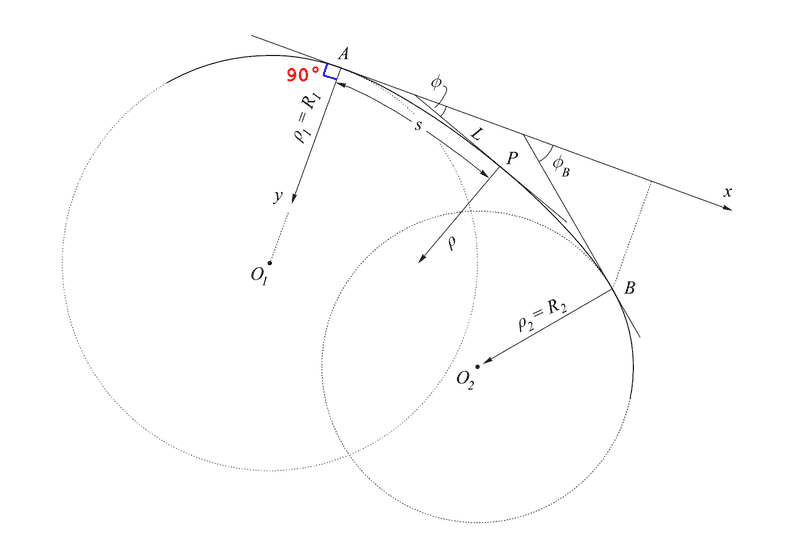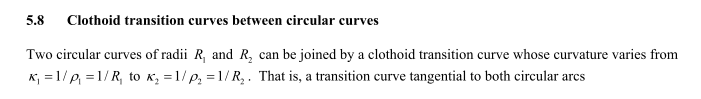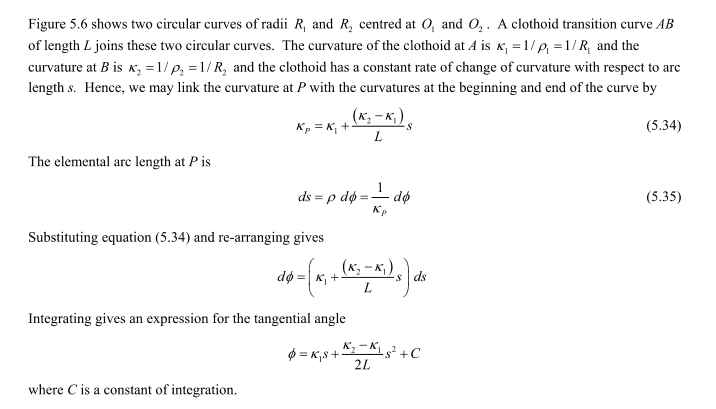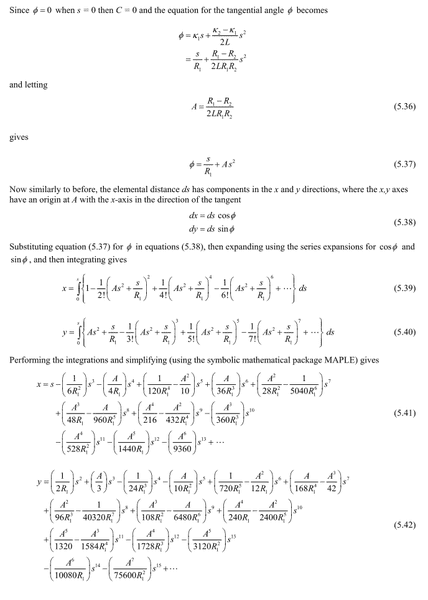(complete document at this link http://www.mygeodesy.id.au/documents/Horizontal Curves.pdf prof. R.E.Deakin)

## The Attempt at a Solution

A iterative solution is using the above equations and change L to find desidered distance of two circle...
but Analitical solution is possible ?
many thanks

P.S. move post here, if I understood well, it was a hint